# 1384 and Level 6

Guessing and checking can be so frustrating! If instead, you study the clues to find a logical place to start this puzzle, you are more likely to be able to find the one and only solution.Print the puzzles or type the solution in this excel file: 10 Factors 1373-1388

Here are a few facts about the number 1384:

• 1384 is a composite number.
• Prime factorization: 1384 = 2 × 2 × 2 × 173, which can be written 1384 = 2³ × 173
• 1384 has at least one exponent greater than 1 in its prime factorization so √1384 can be simplified. Taking the factor pair from the factor pair table below with the largest square number factor, we get √1384 = (√4)(√346) = 2√346
• The exponents in the prime factorization are 3 and 1. Adding one to each exponent and multiplying we get (3 + 1)(1 + 1) = 4 × 2 = 8. Therefore 1384 has exactly 8 factors.
• The factors of 1384 are outlined with their factor pairs in the graphic below.1384 is the hypotenuse of a Pythagorean triple:
416-1320-1384 which is 8 times (52-165-173)

# 1363 and Level 6

The common factors of 60 and 30 allowed in the puzzle are 5, 6, and 10. Which one is the logical choice? Look at the other clues in the puzzle and you should be able to eliminate two of the choices.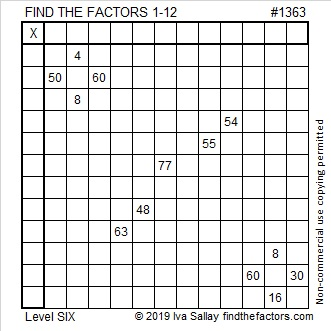Print the puzzles or type the solution in this excel file: 12 Factors 1357-1365

Here are a few thoughts about the puzzle number, 1363:

• 1363 is a composite number.
• Prime factorization: 1363 = 29 × 47
• 1363 has no exponents greater than 1 in its prime factorization, so √1363 cannot be simplified.
• The exponents in the prime factorization are 1, and 1. Adding one to each exponent and multiplying we get (1 + 1)(1 + 1) = 2 × 2 = 4. Therefore 1363 has exactly 4 factors.
• The factors of 1363 are outlined with their factor pair partners in the graphic below.1363 is the hypotenuse of a Pythagorean triple:
940-987-1363 which is (20-21-29) times 47

# 1355 I Can Solve This Puzzle, Can You?

Level 6 puzzles are a little tricky because there is more than one possible common factor for every set of clues on the same row or column.

You can still solve it using logic. I can solve it. Can you?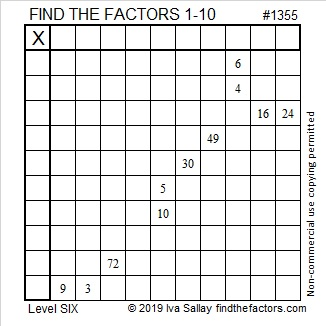Print the puzzles or type the solution in this excel file: 10 Factors 1347-1356

If you need some logical hints, the video below will be helpful:

Now I’ll share some information about the puzzle number, 1355:

• 1355 is a composite number.
• Prime factorization: 1355 = 5 × 271
• 1355 has no exponents greater than 1 in its prime factorization, so √1355 cannot be simplified.
• The exponents in the prime factorization are 1, and 1. Adding one to each exponent and multiplying we get (1 + 1)(1 + 1) = 2 × 2 = 4. Therefore 1355 has exactly 4 factors.
• The factors of 1355 are outlined with their factor pair partners in the graphic below.1355 is the hypotenuse of a Pythagorean triple:
813-1084-1355 which is (3-4-5) times 271

# 1339 Written Eligible Factor Pairs Can Reveal the Needed Logic

The logic needed for this particular level 6 puzzle is VERY complicated so I wrote out the eligible factor pairs of all the clues for you.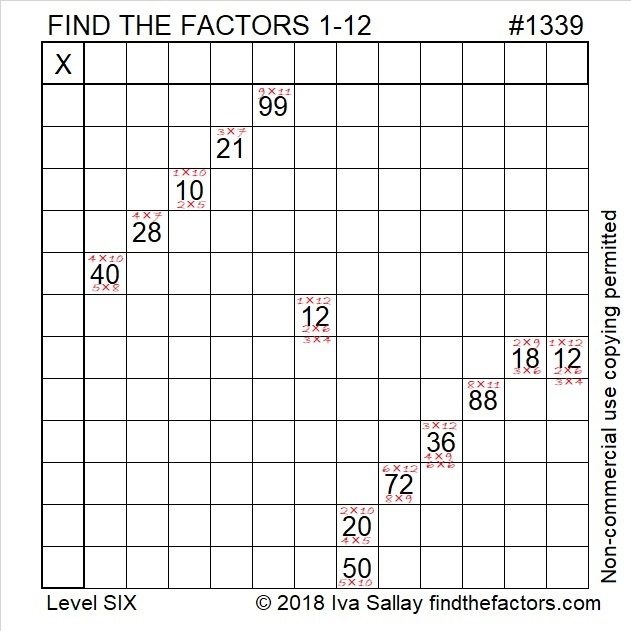Or you can start from scratch:Print the puzzles or type the solution in this excel file: 12 factors 1333-1341

Here are a few facts about the puzzle number, 1339:

• 1339 is a composite number.
• Prime factorization: 1339 = 13 × 103
• 1339 has no exponents greater than 1 in its prime factorization, so √1339 cannot be simplified.
• The exponents in the prime factorization are 1, and 1. Adding one to each exponent and multiplying we get (1 + 1)(1 + 1) = 2 × 2 = 4. Therefore 1339 has exactly 4 factors.
• The factors of 1339 are outlined with their factor pairs in the graphic below.1339 is the hypotenuse of a Pythagorean triple:
515-1236-1339 which is (5-12-13) times 103

# 1329 Flight Plans

Many people fly home or away from home for the holidays. Here’s a puzzle to occupy some of your time while you’re in flight.

Print the puzzles or type the solution in this excel file:10-factors-1321-1332

Now I’ll write a little bit about the number 1329:

• 1329 is a composite number.
• Prime factorization: 1329 = 3 × 443
• The exponents in the prime factorization are 1 and 1. Adding one to each and multiplying we get (1 + 1)(1 + 1) = 2 × 2 = 4. Therefore 1329 has exactly 4 factors.
• Factors of 1329: 1, 3, 443, 1329
• Factor pairs: 1329 = 1 × 1329 or 3 × 443
• 1329 has no square factors that allow its square root to be simplified. √1329 ≈ 36.45545

1329 is divisible by 3 because it is made with three consecutive numbers (1, 2, 3) and 9, a number divisible by 3.

1329 looks interesting in some other bases:
It’s 929 in BASE 12,
369 in BASE 20
234 in BASE 25

# 1320 Christmas Factor Trees

Regardless of its size, an evergreen tree is a mighty symbol at Christmastime. Today’s factoring puzzle features a couple of relatively small Christmas trees, but don’t think for even one minute that these little trees make for an easy puzzle. It’s a level 6 puzzle so there are several places that the clues might trick you. Use logic through the entire process, and you should be able to solve it!

Print the puzzles or type the solution in this excel file: 12 factors 1311-1319

That factoring puzzle has nothing to do with the factors of 1320. In case you are looking for factor trees for the number 1320, here are a few of the MANY possible ones:

Here are some facts about the number 1320:

• 1320 is a composite number.
• Prime factorization: 1320 = 2 × 2 × 2 × 3 × 5 × 11, which can be written 1320 = 2³ × 3 × 5 × 11.
• The exponents in the prime factorization are 3, 1, 1, and 1. Adding one to each and multiplying we get (3 + 1)(1 + 1)(1 + 1) )(1 + 1) = 4 × 2 × 2 × 2 = 32. Therefore 1320 has exactly 32 factors.
• Factors of 1320: 1, 2, 3, 4, 5, 6, 8, 10, 11, 12, 15, 20, 22, 24, 30, 33, 40, 44, 55, 60, 66, 88, 110, 120, 132, 165, 220, 264, 330, 440, 660, 1320
• Factor pairs: 1320 = 1 × 1320, 2 × 660, 3 × 440, 4 × 330, 5 × 264, 6 × 220, 8 × 165, 10 × 132, 11 × 120, 12 × 110, 15 × 88, 20 × 66, 22 × 60, 24 × 55, 30 × 44 or 33 × 40
• Taking the factor pair with the largest square number factor, we get √1320 = (√4)(√330) = 2√330 ≈ 36.331804

1320 is the sum of consecutive primes FOUR different ways:
It is the sum of the eighteen primes from 37 to 109,
107 + 109 + 113 + 127 + 131 + 137 + 139 + 149 + 151 + 157 = 1320,
149 + 151 + 157 + 163 + 167 + 173 + 179 + 181 = 1320, and
659 + 661 = 1320

1320 is the hypotenuse of a Pythagorean triple:
792-1056-1320 which is (3-4-5) times 264

# 1308 and Level 6

Can you find the factors that will turn this puzzle into a multiplication table? It’s a level 6 so you might find it to be a challenge. Use logic. Don’t guess and check.Print the puzzles or type the solution in this excel file: 10-factors-1302-1310

Here are a few facts about the puzzle number, 1308:

• 1308 is a composite number.
• Prime factorization: 1308 = 2 × 2 × 3 × 109, which can be written 1308 = 2² × 3 × 109
• The exponents in the prime factorization are 2, 1, and 1. Adding one to each and multiplying we get (2 + 1)(1 + 1)(1 + 1) = 3 × 2 × 2 = 12. Therefore 1308 has exactly 12 factors.
• Factors of 1308: 1, 2, 3, 4, 6, 12, 109, 218, 327, 436, 654, 1308
• Factor pairs: 1308 = 1 × 1308, 2 × 654, 3 × 436, 4 × 327, 6 × 218, or 12 × 109
• Taking the factor pair with the largest square number factor, we get √1308 = (√4)(√327) = 2√327 ≈ 36.16628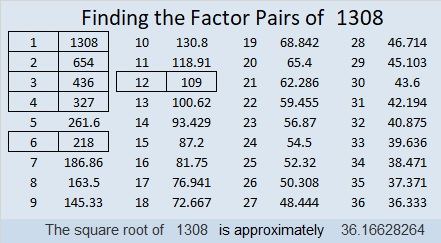1308 is the hypotenuse of a Pythagorean triple:
720-1092-1308 which is 12 times (60-91-109)

As shown in their factor trees below, 1308, 1309, 1310, and 1311 each have three distinct prime numbers in their prime factorizations. They are the smallest set of four consecutive numbers with the same number of prime factors. Thank you Stetson.edu for alerting me to that fact.# 1294 and Level 6

You will need to use multiplication facts from 1 to 12, logic, and perseverance to solve this tricky level 6 puzzle. Good luck!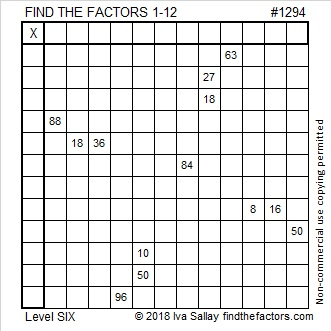Print the puzzles or type the solution in this excel file: 12 factors 1289-1299

Here are some facts about the number 1294:

• 1294 is a composite number.
• Prime factorization: 1294 = 2 × 647
• The exponents in the prime factorization are 1 and 1. Adding one to each and multiplying we get (1 + 1)(1 + 1) = 2 × 2 = 4. Therefore 1294 has exactly 4 factors.
• Factors of 1294: 1, 2, 647, 1294
• Factor pairs: 1294 = 1 × 1294 or 2 × 647
• 1294 has no square factors that allow its square root to be simplified. √1294 ≈ 35.972211294 is also the sum of the twenty prime numbers from 23 to 107.

# 1286 and Level 6

This level 6 puzzle can be solved by using logic and basic knowledge of the multiplication table. Stay focused, and you will get it done!Print the puzzles or type the solution in this excel file: 10-factors-1281-1288

Here are a few facts about the number 1286:

• 1286 is a composite number.
• Prime factorization: 1286 = 2 × 643
• The exponents in the prime factorization are 1 and 1. Adding one to each and multiplying we get (1 + 1)(1 + 1) = 2 × 2 = 4. Therefore 1286 has exactly 4 factors.
• Factors of 1286: 1, 2, 643, 1286
• Factor pairs: 1286 = 1 × 1286 or 2 × 643
• 1286 has no square factors that allow its square root to be simplified. √1286 ≈ 35.86084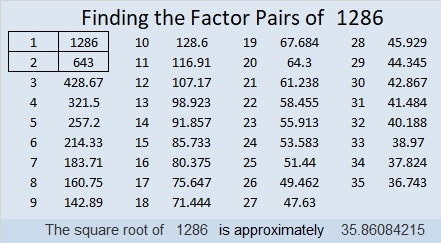1286 is also the sum of six consecutive prime numbers:
197 + 199 + 211 + 223 + 227 + 229 = 1286

# 1280 and Level 6

To me, today’s level 6 puzzle looks a little like a puppy dog. If you know or use a multiplication table, then with proper training, finding the factors of this puzzle will be no problem.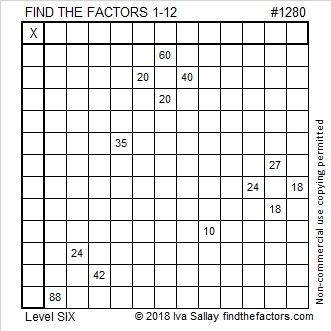Print the puzzles or type the solution in this excel file: 12 factors 1271-1280

I’d like to tell you a little about the number 1280:

• 1280 is a composite number.
• Prime factorization: 1280 = 2 × 2 × 2 × 2 × 2 × 2 × 2 × 2 × 5, which can be written 1280 = 2⁸ × 5
• The exponents in the prime factorization are 8 and 1. Adding one to each and multiplying we get (8 + 1)(1 + 1) = 9 × 2 = 18. Therefore 1280 has exactly 18 factors.
• Factors of 1280: 1, 2, 4, 5, 8, 10, 16, 20, 32, 40, 64, 80, 128, 160, 256, 320, 640, 1280
• Factor pairs: 1280 = 1 × 1280, 2 × 640, 4 × 320, 5 × 256, 8 × 160, 10 × 128, 16 × 80, 20 × 64, or 32 × 40
• Taking the factor pair with the largest square number factor, we get √1280 = (√256)(√5) = 16√5 ≈ 35.77709.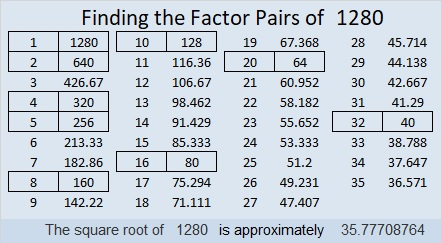1280 is the sum of the fourteen prime numbers from 61 to 127. Do you know what those prime numbers are?

32² + 16² = 1280

1280 is the hypotenuse of a Pythagorean triple:
768-1024-1280 which is (3-4-5) times 256
That triple can also be calculated from 32² – 16², 2(32)(16), 32² + 16²

Since 1280 is the 5th multiple of 256, I would expect that a number close to 1280 would be the 500th number whose square root could be simplified. That number was 1275, just five numbers ago.